﻿ PipeLay > Software Components & Operation > Environment, Motion & Loading > Environment Components > Wave > Wave - Random Sea

# Wave - Random Sea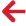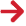Of the 25 Wave Type options, 18 relate to random seas. PipeLay has 5 wave spectrum options, namely Pierson-Moskowitz, JONSWAP, and User-Spectrum. As noted previously, all of the spectrum options are present in a number of Wave Type combinations.

Some of these combinations differ in the algorithm they use to discretise the wave spectrum. In a random sea analysis, PipeLay discretises the spectrum you specify into discrete harmonics, and uses these to generate a random time history of water surface elevation using a Monte Carlo simulation technique. This provides the basic dynamic random excitation on the structure. PipeLay provides two different algorithms for discretising a wave spectrum. These are now described first, before proceeding to looking at all of the random sea options in detail.

Spectrum Discretisation

The two PipeLay spectrum discretisation options are termed the Equal Area and the Geometric Progression algorithms.

If you nominate the first option, Equal Area, the random wave spectrum is discretised into component harmonics by dividing the area under the spectrum into segments of equal area. The area of each segment is the total area under the spectrum divided by the number of harmonics you specify when inputting your wave spectrum data and each area then defines a single harmonic in the discretisation.

This procedure has the disadvantage that it leads to increasingly larger frequency increments at higher frequencies and a poor representation of higher harmonics in the wave elevation time series. PipeLay provides a Max Frequency Increment input (which you also specify as part of your wave spectrum data) to counteract this. If a particular segment requires a frequency increment greater than the maximum you specify, the segment area is continuously halved until the required increment is less than the maximum. In general, this leads to a greater number of harmonics being returned by the discretisation than the value you specify.

With the second option, denoted Geometric Progression, the wave spectrum is discretised into segments based on frequency increments that form a geometric progression. The procedure adopted is as follows. The total area under the wave spectrum, up to a cut-off frequency you specify, is found, and the frequency that corresponds to the first 1% of the area under the wave spectrum is identified. Next, this frequency is multiplied by a constant factor to give the next frequency in the series. The area between these two frequencies is taken as the first discrete segment of the wave spectrum. The next frequency in the series is found by again multiplying the preceding frequency by the constant factor, and the area between these two frequencies is taken as the next discrete segment of the wave spectrum. This procedure is repeated until the frequency corresponding to the first 99% of the area under the wave spectrum is reached. The constant factor which multiplies successive frequencies is given by (1 + Geometric Progression Factor), this parameter being a user input.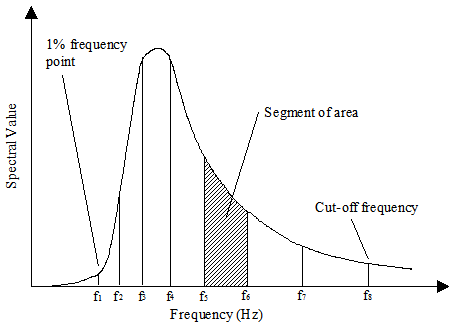Geometric Progression Wave Spectrum Discretisation

The figure above shows an example of geometric progression wave spectrum discretisation. Each segment of area of the wave spectrum is defined by the frequencies at the start and end of the segment, fn and fn+1, where: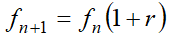r is the Geometric Progression Factor you input. Each segment of area defines a single harmonic in the discretisation, with the frequency of the harmonic for a particular segment given by: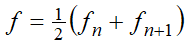PipeLay uses a default value of 0.02 for the geometric progression factor, which is sufficient in most cases. The smaller the value used, the greater will be the number of harmonics in the resulting discretisation, and the longer will be the repeat time of the resulting wave elevation time series. Note that the figure above is illustrative only, generally a much larger number of harmonics should be produced by the wave spectrum discretisation.

## Dedicated Wave Spectra

PipeLay provides 4 different types of dedicated wave spectra that are based on well established wave theory used in the offshore industry. These include Pierson-Moskowitz, JONSWAP, andRefer to the 'Wave - Random Sea Spectra' article for more information.

## User Spectrum

For complete generality, PipeLay also provides an option for you to input your wave spectrum directly, as a series of (frequency, spectral ordinate) data pairs; this is the user-specified spectrum option. There are again two Wave Type options for including a user-specified spectrum in your dynamic analysis, corresponding to the two discretisation algorithms:

User Specified - Equal Area Discretisation

User Specified - Geometric Progression Discretisation

The specification of data for this option is in two parts. Firstly, in the Wave Data dialog you input discretisation-specific data only. Obviously the format here depends on the discretisation algorithm you nominate – for example, the figure below shows the format for the equal area discretisation, where the entries are similar to previous spectrum types.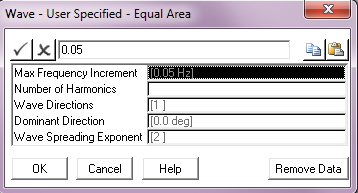User Specified Wave Data; Equal Area Discretisation

Secondly, you must also input the actual spectrum data pairs, which you do using the Wave component User Spectrum dialog; this is naturally enabled only when you choose either of the user specified spectrum options. The format of this dialog is shown in the figure below. The specification is straight-forward. You use one line per pair of values, repeated as often as necessary to complete define your spectrum.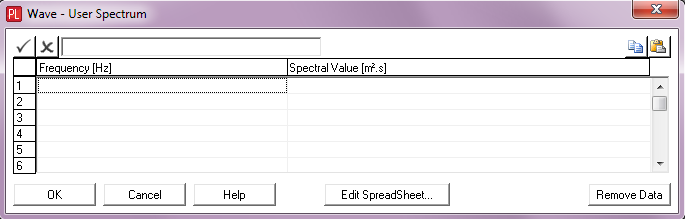Input of User Specified Spectrum

## Random Seed

When you choose any of the PipeLay random sea options, the Random Seed button on the Wave component is enabled. This allows you to specify a seed value for the random number generator that assigns random phases to wave components when discretising a wave spectrum. Unless you specify otherwise, PipeLay always uses the same seed value, so that every time you discretise the same spectrum with the same discretisation parameters, you get the same sequence of random phases and consequently the same time history of water surface elevation. You may want to change this, so that you get a different set of phases and so a different wave elevation time history from the same spectrum and discretisation parameters (other than the seed). This can be done by specifying a different seed value here.

The dialog you get when you click on is shown in the figure below.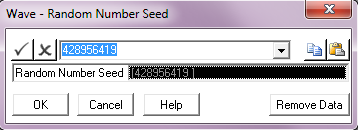Input of Random Seed

PipeLay uses a Multiplicative Congruential Generator to generate a sequence of random phases. For best results, the random number seed for this should be a large (and preferably prime) number. You can enter your own value for the random number seed in the dialog box shown above. Alternatively, you can select a seed value from the drop-down list provided. If you do not specify a seed value, PipeLay uses the first value shown in the drop-down list above by default.